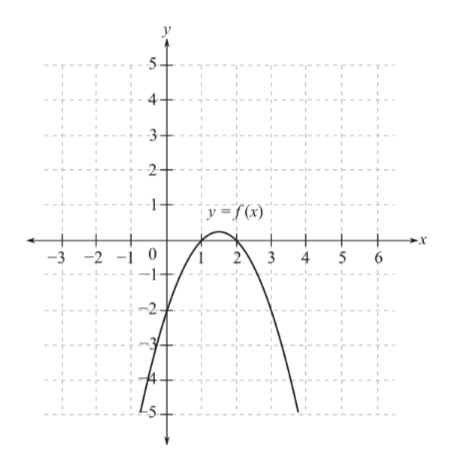In my precalc 11 class this week, I learned about graphing, and how it can relate to a quadratic equation or function. A quadratic function is in the form y=ax² + bx + c

These are all quadratic equations: Even if they don’t look like it at the start, they can be moved around into the correct form.These graphs in curved shapes are called parabolas:When an equation creates a graph in a parabola, it is a quadratic function. Parabolas have a vertex, which is either the highest or lowest point. If it is the highest point on the graph, it is called the maximum point. If it is at the bottom, it is the minimum point. Here are some examples: